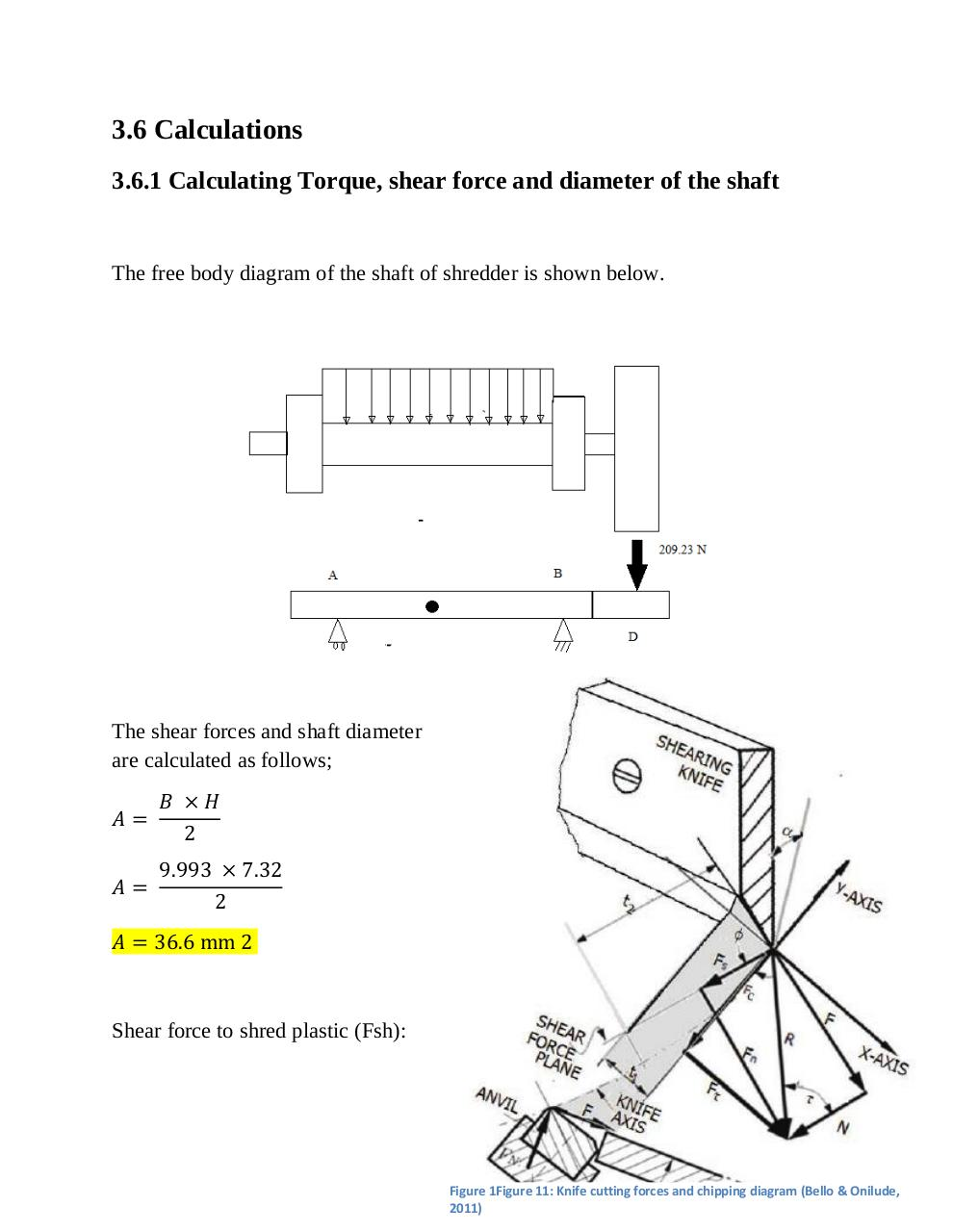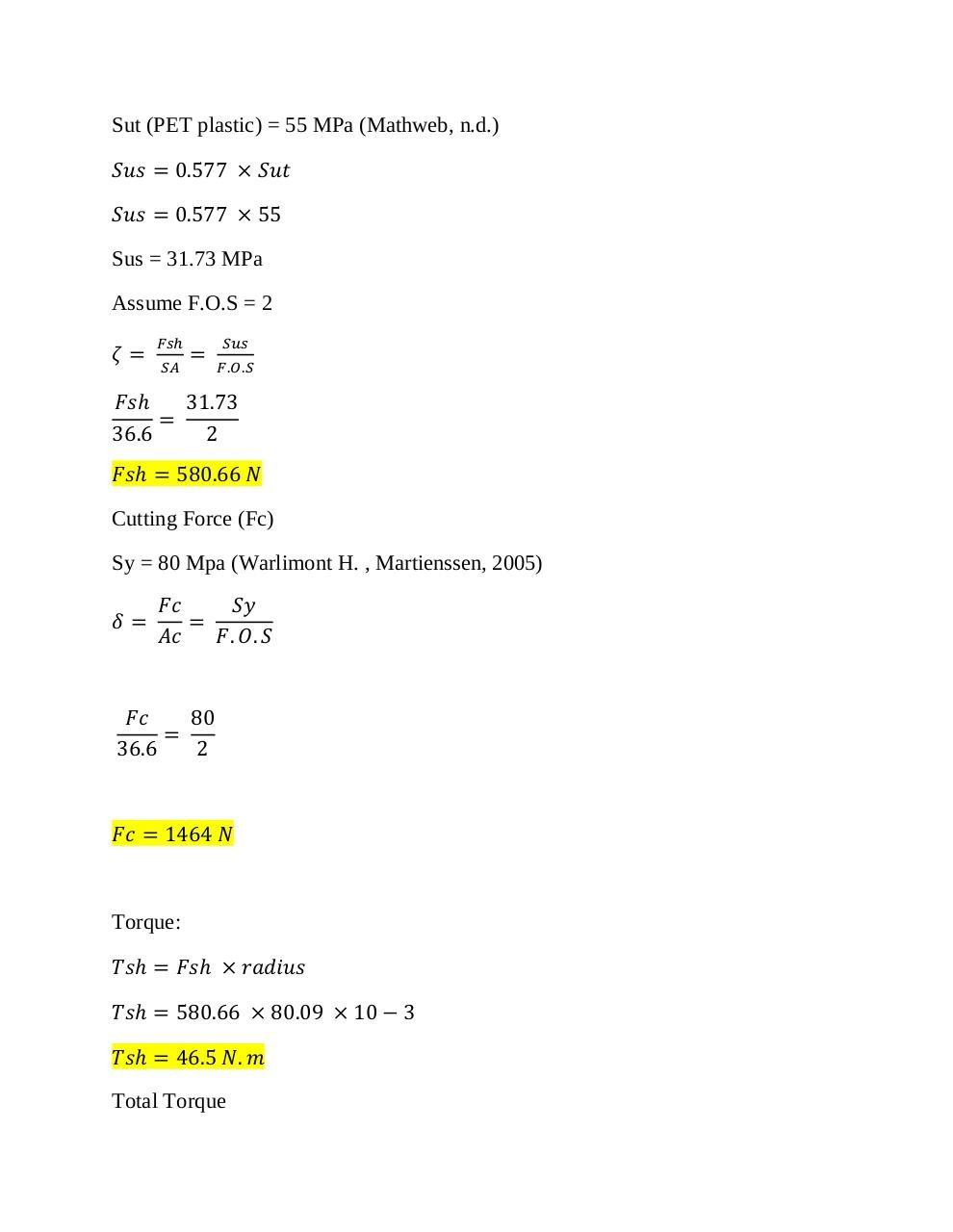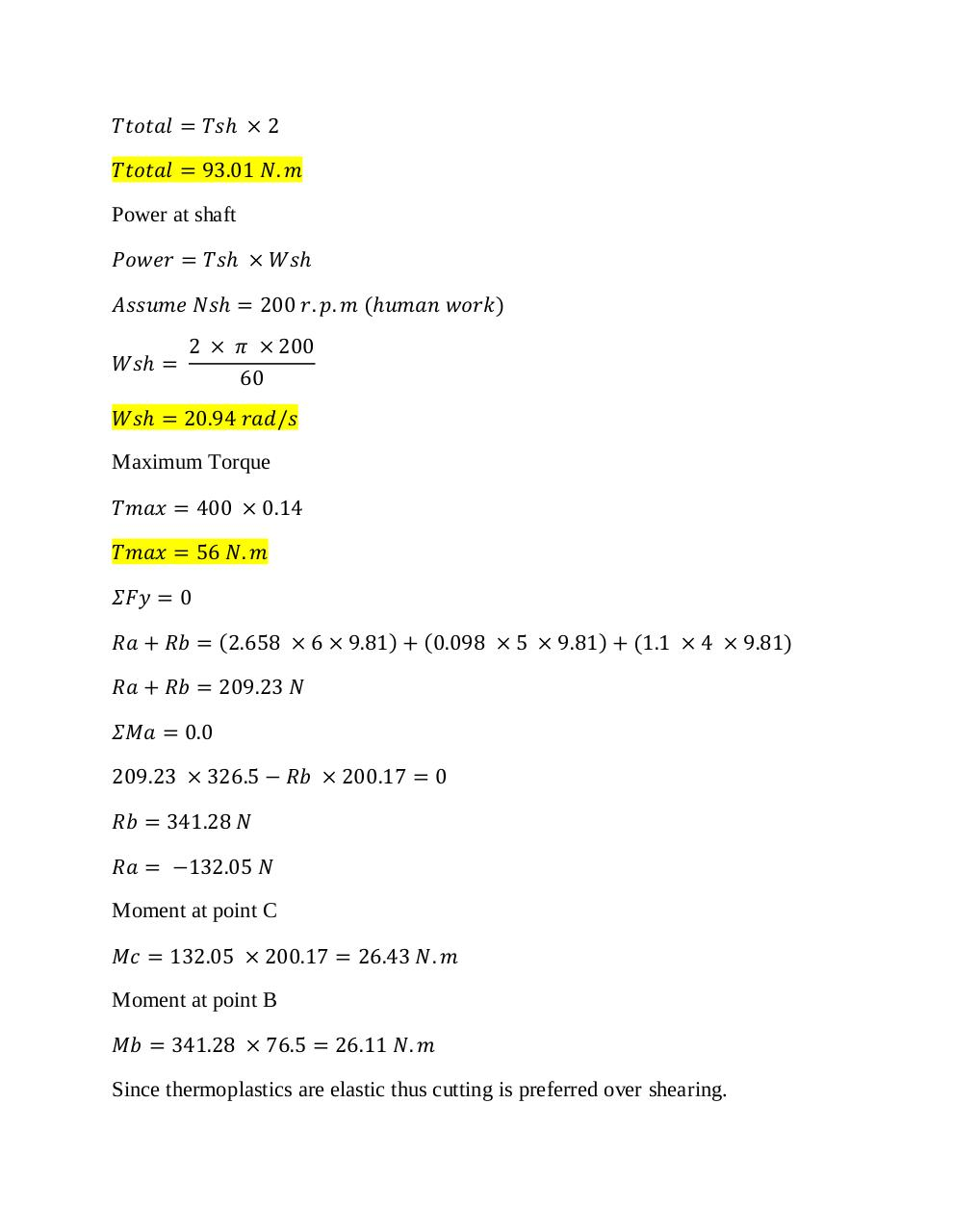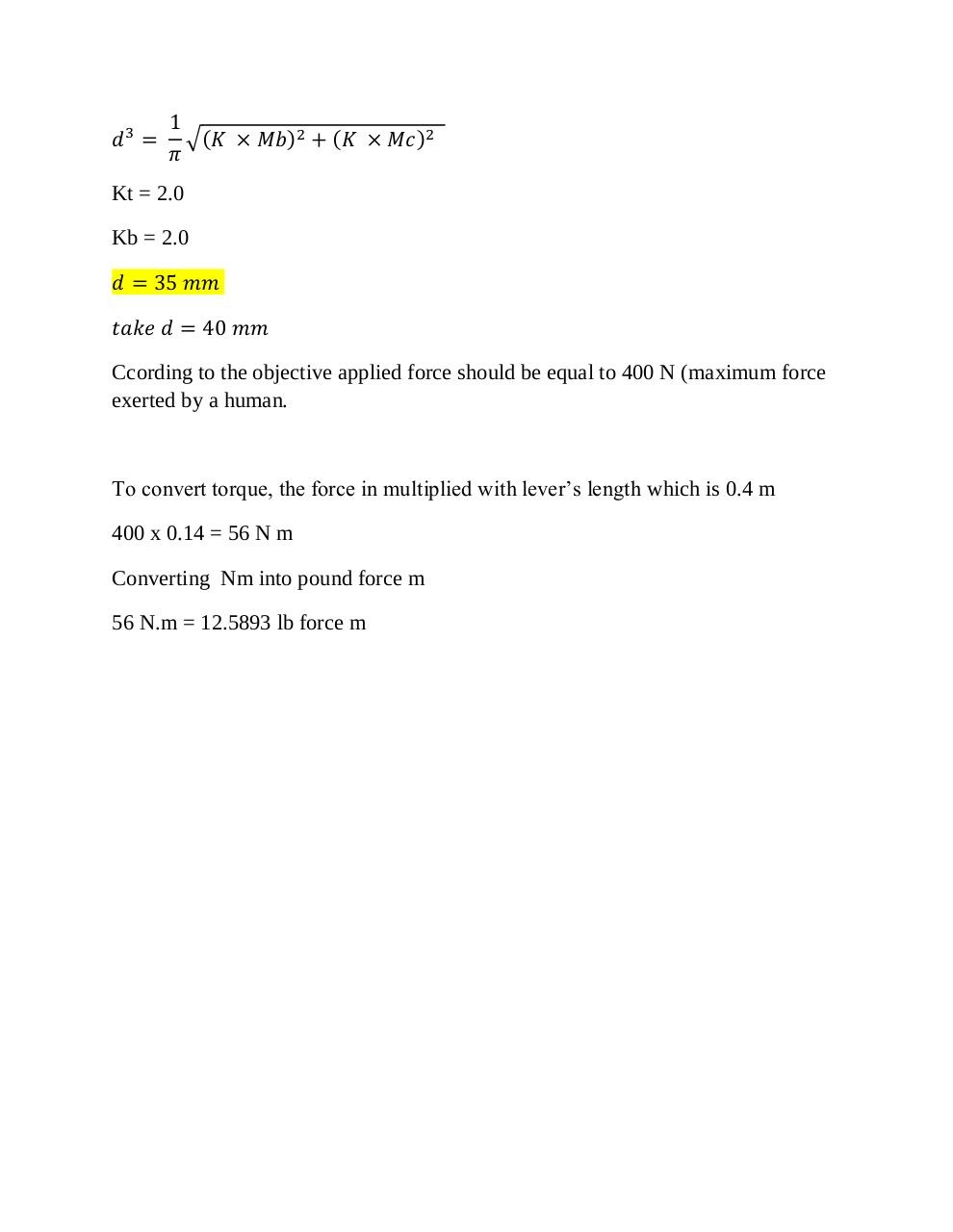# shredder calculations .pdf

### File information

Original filename: shredder calculations.pdf

This PDF 1.5 document has been generated by MicrosoftÂ® Word 2010, and has been sent on pdf-archive.com on 01/05/2018 at 15:26, from IP address 60.50.x.x. The current document download page has been viewed 7738 times.
File size: 581 KB (4 pages).
Privacy: public file

shredder calculations.pdf (PDF, 581 KB)

### Document preview

3.6 Calculations
3.6.1 Calculating Torque, shear force and diameter of the shaft

The free body diagram of the shaft of shredder is shown below.

The shear forces and shaft diameter
are calculated as follows;

Shear force to shred plastic (Fsh):

Figure 1Figure 11: Knife cutting forces and chipping diagram (Bello &amp; Onilude,
2011)

Sut (PET plastic) = 55 MPa (Mathweb, n.d.)

Sus = 31.73 MPa
Assume F.O.S = 2

Cutting Force (Fc)
Sy = 80 Mpa (Warlimont H. , Martienssen, 2005)

Torque:

Total Torque

Power at shaft

Maximum Torque

Moment at point C

Moment at point B

Since thermoplastics are elastic thus cutting is preferred over shearing.

Kt = 2.0
Kb = 2.0

Ccording to the objective applied force should be equal to 400 N (maximum force
exerted by a human.

To convert torque, the force in multiplied with lever’s length which is 0.4 m
400 x 0.14 = 56 N m
Converting Nm into pound force m
56 N.m = 12.5893 lb force m#### HTML Code

Copy the following HTML code to share your document on a Website or Blog

#### QR Code### Related keywords